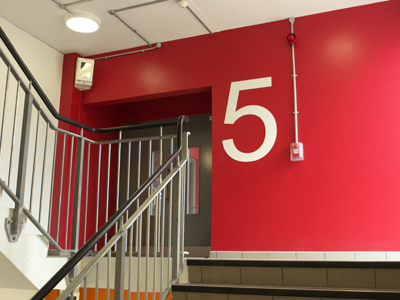1 x 5 = 5.

# The 05 Times Table

The five times table is one of the easier times tables to learn - and learn it you must for KS3 Maths. One trick that might help you is to multiply the numbers by 10 and then halve them. For example 7 x 5. If you multiply 7 x 10, you get 70. Half of 70 is 35. Therefore 7 x 5 must equal 35 - simple, isn't it?

Five is quite a common number. The Olympic symbol has five rings. Basketball is played with teams of five players. We haves five senses: smell, touch, sight, hearing and taste. There are five elements in Chinese philosophy: water, wood, fire, metal and earth. There are five vowels in the English alphabet: a, e, i, o and u. And the lowest value note is a £5 note.

This may be one of the easiest times tables but don't rush through the questions. A moment of carelessness may lead you to click on the wrong answer by mistake. As with all tests, read the questions properly and choose your answers carefully.

1.
What is 2 x 5
10
25
20
15
To multiply a number by 2 just double it
2.
What is 5 x 5
26
25
20
24
5 is the square root of 25
3.
What is 3 x 5
15
18
35
25
A rugby union team has 15 players
4.
What is 7 x 5
55
35
45
25
Remember, to multiply any number by 5, first times it by 10 and then halve your answer
5.
What is 6 x 5
33
35
30
25
4 months - April, June, September and November - have 30 days
6.
What is 9 x 5
40
45
25
35
To solve this question you could first multiply 5 x 10 and then subtract 5
7.
What is 8 x 5
36
42
40
38
There are 40 spaces on a Monopoly game board
8.
What is 10 x 5
50
40
60
20
To multiply any number by 10, simply put a zero on its end
9.
What is 1 x 5
246
247
5
245
Any number multiplied by one remains unchanged
10.
What is 4 x 5
24
30
16
20
Another name for the number 20 is a 'score'
Author:  Frank Evans